# Formula Columns

In addition to table columns containing simple values, such as numeric or text types, TeamDesk allows users to create columns with formula values. The value for such columns is determined as a result of configuration or calculation by predefined formulas.

Formula-defined columns are created in the same way as columns of ordinary types (see the Creating New Columns section). For columns that contain formulas, you may use the following data types:

• Formula - Text: This type of column allows formulas that provide a text result. The formula may contain a text literal, variable, or references to columns that store text values.

• Formula - Number: This type allows formulas that provide a numeric result, whether integer, decimal, positive, or negative. The formula may contain actual numbers (literals), references to columns that store numeric values, or variables that should be converted first using the ToNumber function. For example, to calculate age using the birthdate value, insert the following formula into the formula-numeric column: `Year(Today()) - Year([Birthdate]) - If(DayOfYear(Today()) < DayOfYear([Birthdate]), 1, 0)`

• Formula - Date: This type allows formulas that provide a date. If in the formula you use a literal or variable, convert it into a date first using the ToDate function. To retrieve today’s date, use the Today function.

• Formula - Time: This type allows formulas that display the time of a day. The result may be created by retrieving time from a Timestamp column or by constructing time with the help of literal values or column references.

• Formula - Duration: This type allows formulas that calculate a certain period of time. To calculate a duration formula, you may choose from the list of Duration functions. In addition, a duration formula may be composed by subtracting one value from another.

• Formula - Checkbox: A checkbox formula always provides a 'Yes' (the checkbox is selected if the expression is true for the record) or 'No' response (the checkbox is not selected if the expression is false).

• Formula - Phone Number: This type allows formulas that create a phone number from the area code, phone number, and possibly an extension. Use text values for formula arguments: literals, variables, or column references.

• Formula - E-mail Address: This type allows formulas used to create an e-mail address either by entering a textual literal in double quotes directly (for example, `"info@mywebsite.com"`) or by combining various column values (for example, `List("@", [Local E-mail Part], [domain])`). Use text values for formula arguments.

• Formula - URL: Formulas for URL can be composed either by entering a textual literal directly (`"www.teamdesk.net"`) or by combining the URL from constituent parts, like variables, literals, column references, and other text values.

• Formula - User: This type of column allows providing names of registered database users. The formula may contain column references or text literals converted into the required data format.

• Formula-Barcode: Any text value can be displayed as a barcode. The formula-barcode column allows generating barcodes that can be inserted into the document and scanned.

• Formula-Location: This type of column options match the Location column. Also, there are a couple of functions you can use with locations.

After a column of a required type has been created, it is necessary to specify the formula itself in the properties of a created column.

To set a new formula:

Click the Setup link at the top right corner of the window, select the table you need.

From the setup menu select `Columns` > `Customize existing columns` link. The system will display the Columns form, click the `Edit` button next to a newly created formula-defined column.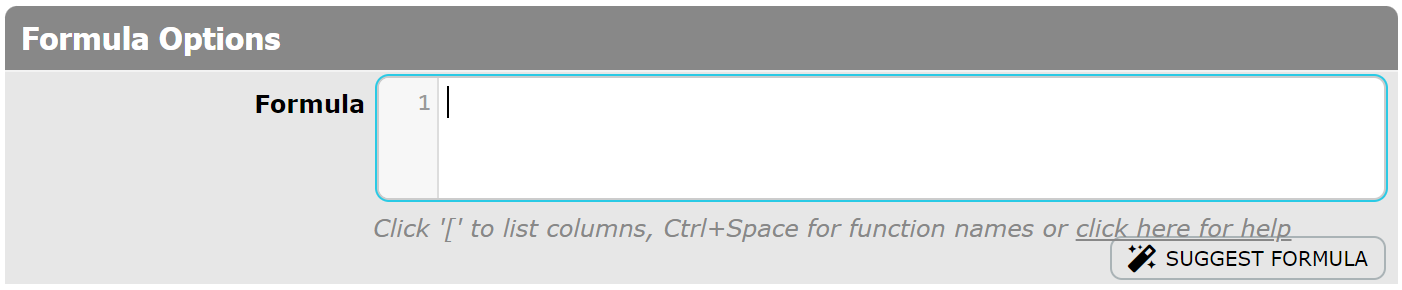In the Formula field, set a new formula according to which the value of a column will be set.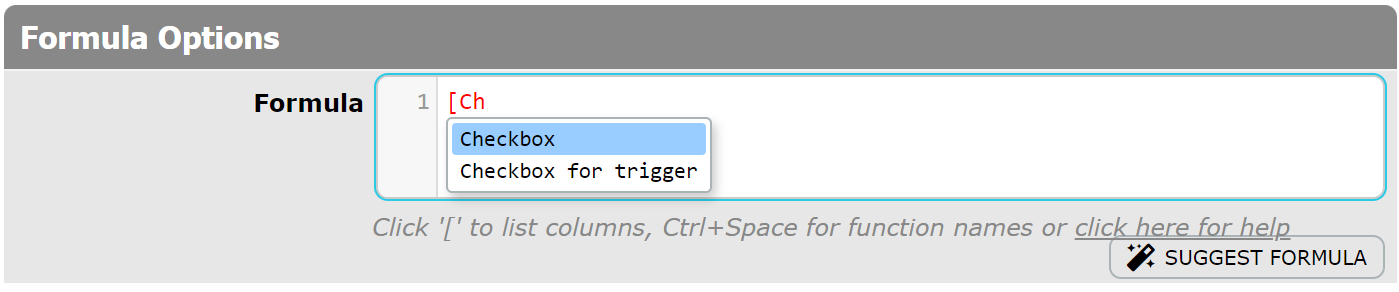Click '[' to list all columns of a table and insert column variables you need. Also, you can type the first letters of the column name after '[' sign, and the columns starting with these letters will be displayed in the dropdown.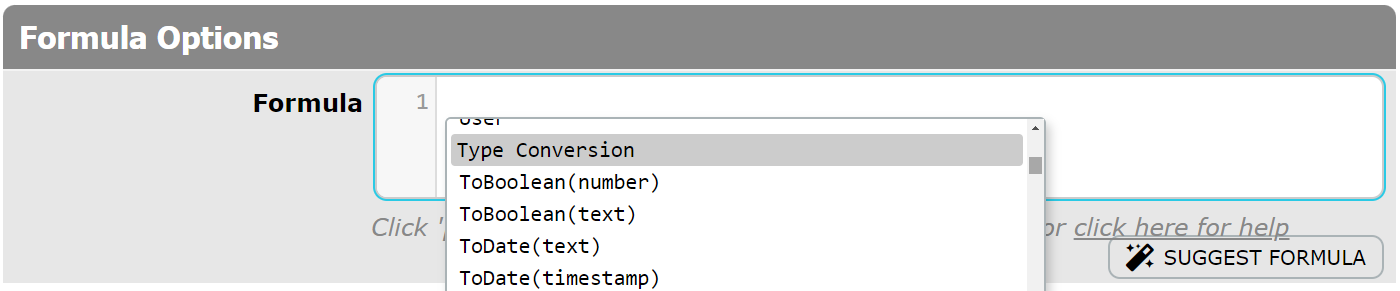Click `Ctrl+Space` to list function names and select functions you need.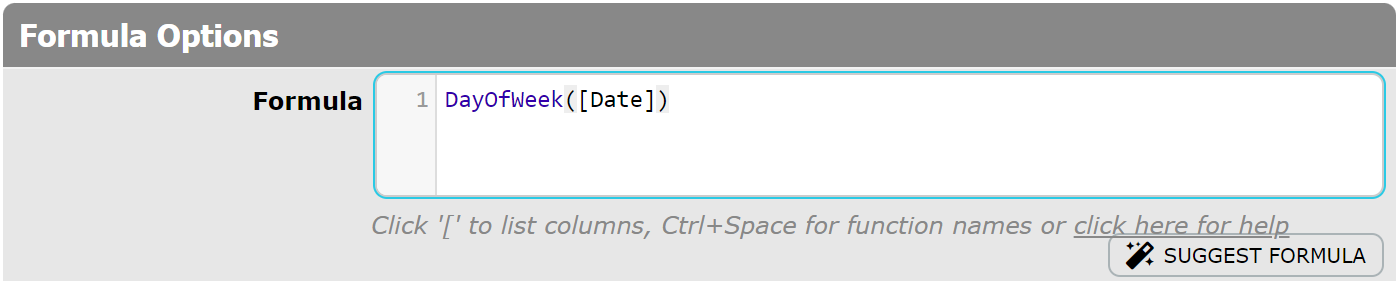If you need information about the Formula Language Reference, use the `click here for help` link under the formula area.

To view a full list of functions used for formula generation, see Formula Language Reference.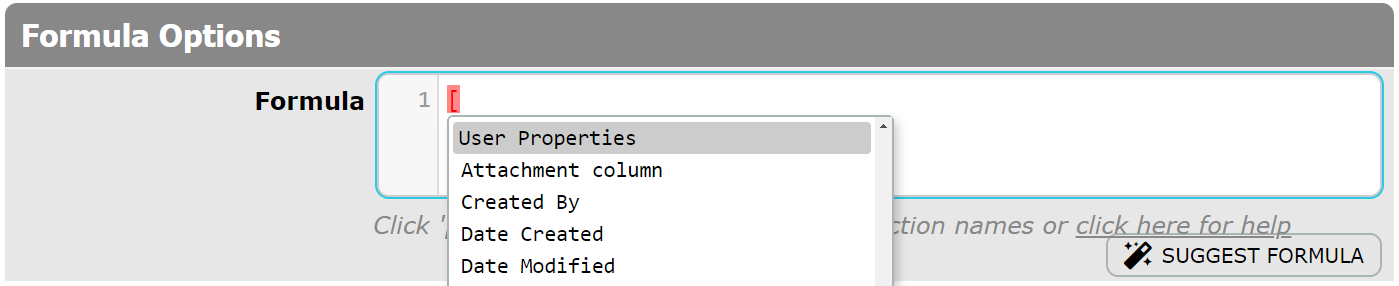Wherever you need information from User Properties related to a current user, you can simply refer to a column of the User Property table by its name. Just click '[' to list all columns of a table and choose a column from the User Properties section. Also, you can type the first letters of the column name after '[' sign, and the columns starting with these letters will be displayed in the dropdown.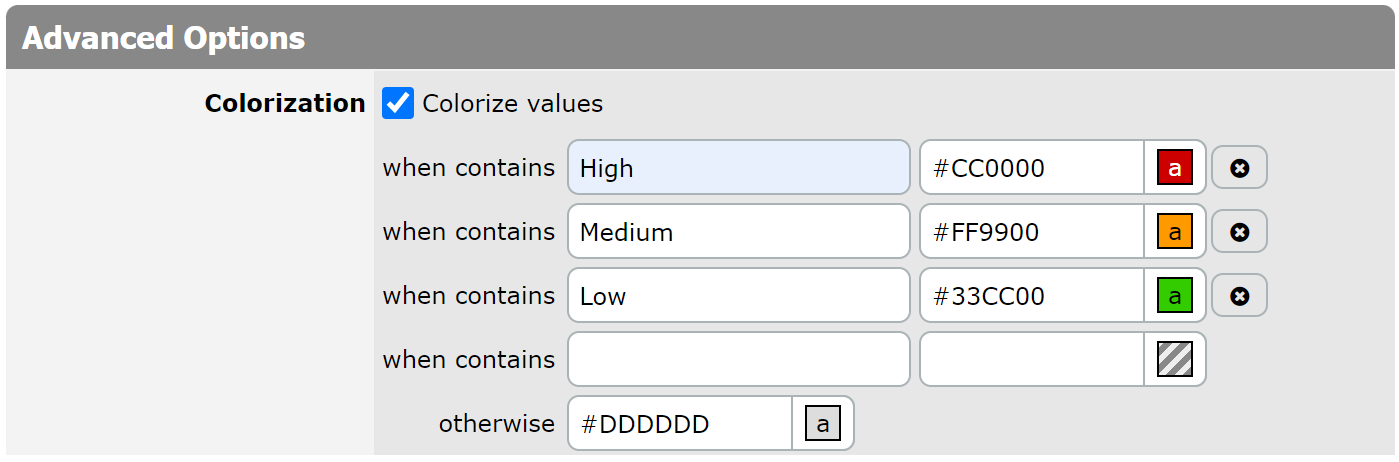You can colorize values stored in the Formula-Text columns. Just use the `Colorization` checkbox displayed in the `Advanced Options`. Colorization is based on whether the value contains certain text. More details are described in the Column Value Colorization section.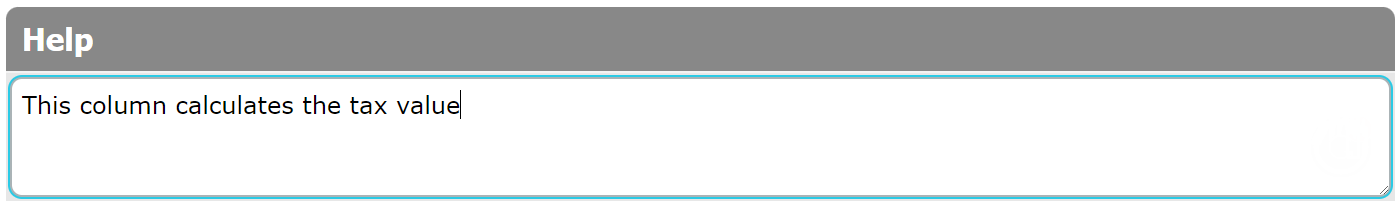The last option is the Help field.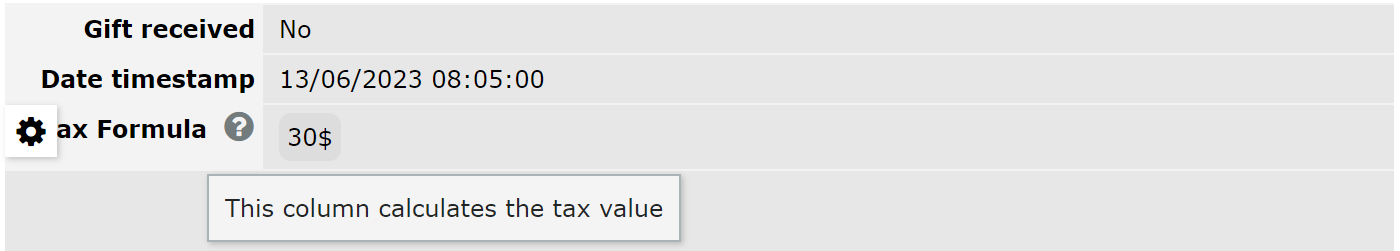When this Help text property is filled in, the question mark is displayed near the field name on the View/Edit form. Hovering over this question mark will display a pop-up with a tip or a help text message to a user.